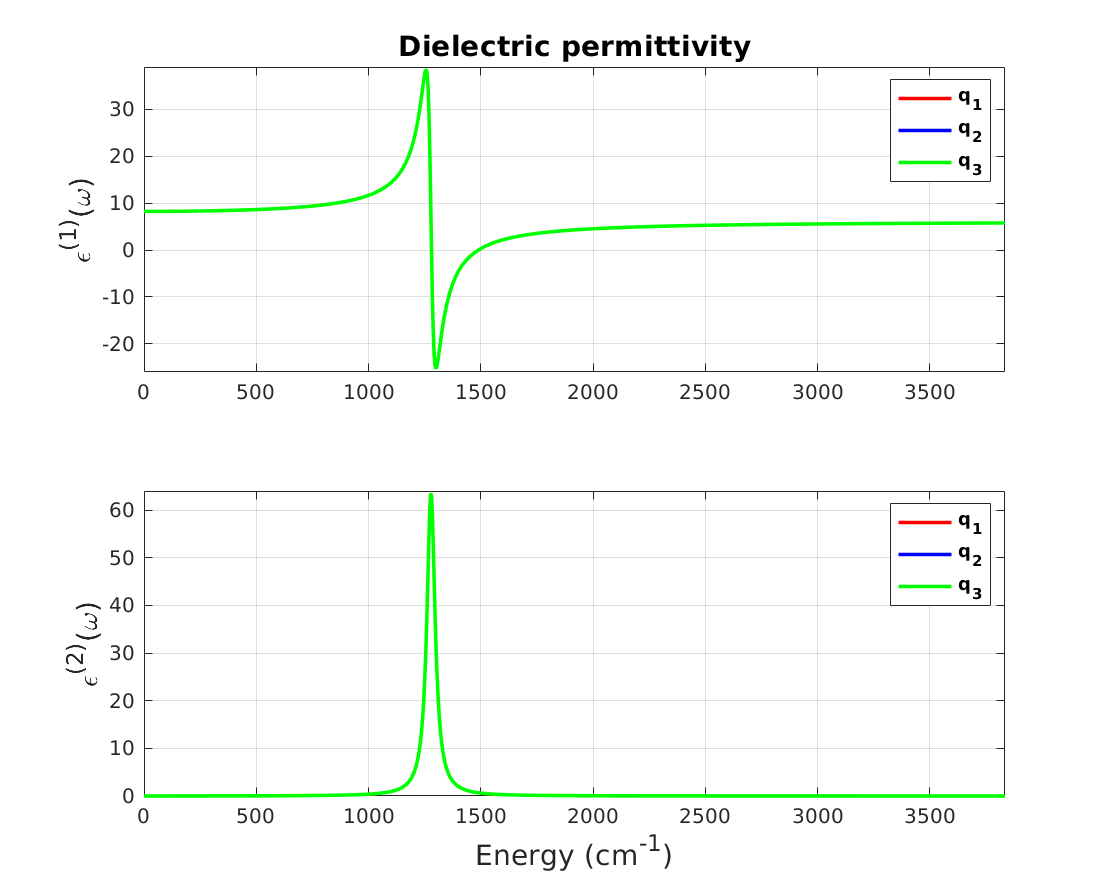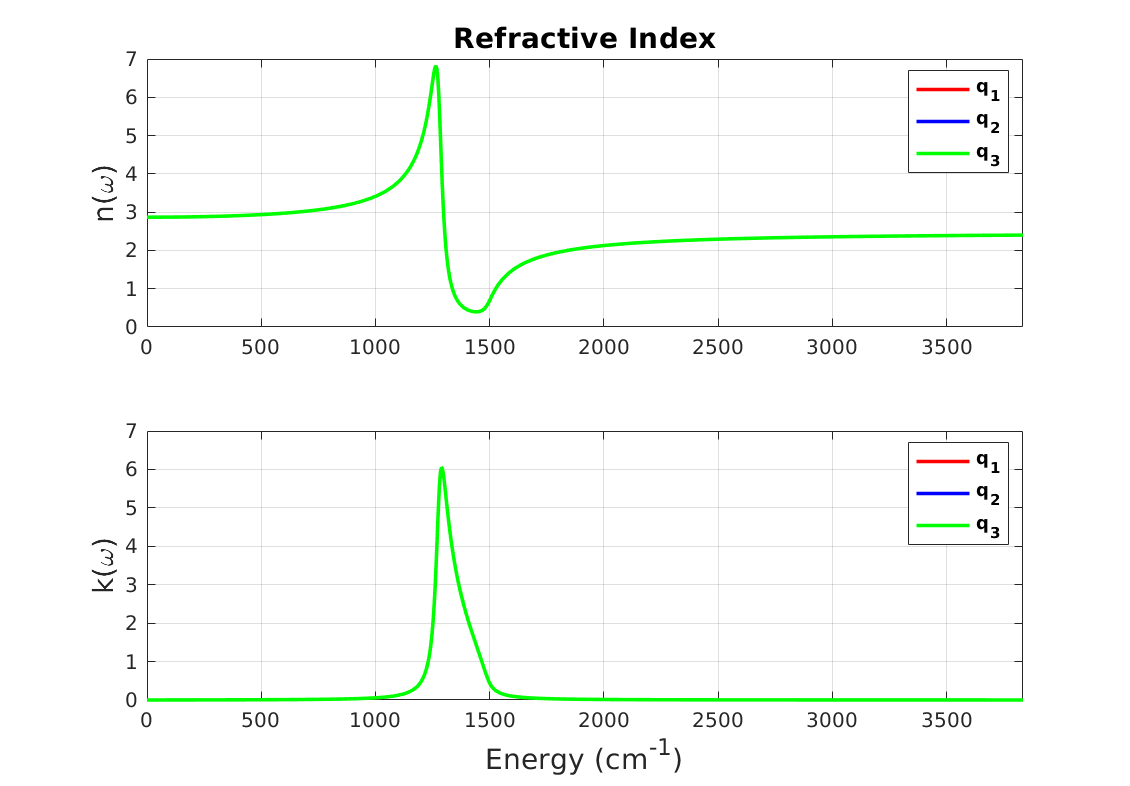# 10.6. Infrared region optical properties

Optical properties at infrared region are determined by phonons at long-wavelength limit, i.e. $$\mathbf{q} \to 0$$. Therefore, atomistic calculation of these properties only requires dynamical matrix at $$\Gamma$$-point, ion-clamped dielectric constant, and Born effect charges [GL97]. Currently RESCU can compute the following properties:

• Complex frequency dependent dielectric permittivity $$\epsilon(\omega)$$, which at $$\omega \to 0$$ returns the static dielectric constant $$\epsilon^{0}$$

• Complex refractive index $$R(\omega)$$

• Infrared intensities, $$I(\omega)$$

• Photon energy loss function due to interaction with phonons

Note

These properties are well-defined for non-metallic systems.

Optical properties generally depend on the angle of interaction of incoming electric field and the surface of material, and in RESCU this direction(s) should be provided as an input by users. This direction is also used to calculate non-analytical effect long-wavelength phonons, LO-TO splitting effect.

Following sample input file can be used to calculate optical properties of Boron nitride. Here we assume that Preparing the ground state data, Ion-clamped dielectric tensor, and Dynamical matrix and Born effective charges sections are already completed.

info.calculationType = 'dfpt-optic'
info.savepath = './results/bn_real_optic'
dfpt.phononData = './results/bn_real_phonon.mat'
dfpt.dielectricData = './results/bn_real_dielectric.mat'
dfpt.qpointCartesian = [1 0 0;1 1 0;1 1 1]
dfpt.opticDamping = 1e-4
dfpt.asr = [1,1,1]


The keywords are explained as

• info.calculationType is a string determining type of the calculation;

• info.savepath points to the file where the output data of calculation are stored;

• dfpt.dielectricData points to the path of the file containing the ion-clamped dielectric tensor (only required for non-metallic systems), i.e. the output of dielectric calculation;

• dfpt.phononData points to the path of the file containing the dynamical matrix and Born effective charges, i.e. the output of phonon calculation;

• dfpt.qpointCartesian a $$N_{direction}\times 3$$ matrix where each row corresponds to a direction of incident electric field for which the optical properties are calculated. By default, RESCU uses the three Cartesian directions;

Note

The direction of incoming electric field can be set by either dfpt.qpointKdirect or dfpt.qpointCartesian. They both accept a $$N_{direction}\times 3$$ matrix where each row represents the direction of an incoming field, expressed in direct or Cartesian coordinates of the reciprocal space, respectively. For example, an incoming photon along the first reciprocal-space lattice vector can be determined by dfpt.qpointKdirect = [1,0,0].

• dfpt.opticDamping damping factor used for Lorentzian broadening of the optical properties in “Hartree” (1 Hartree $$\simeq 6579.7$$ THz). This is a representation of temperature and acts as the damping factor for vibration of atoms as damped harmonic oscillators [GL97].

• dfpt.asr it is a $$1\times 3$$ vector which determines the status of various acoustic sum rules in DFPT calculations. In particular, the first two entries determines if acoustic sum rule and charge neutrality will be enforced to dynamical matrix and Born effective charges, respectively. RESCU assumes that all sum rules are active by default.

RESCU is executed as follows

rescu -i bn_real_optic


The output data can be found in dfpt and optic fields of ./results/bn_real_phonon.mat output file.

>> dfpt

dfpt =

struct with fields:

BornEC: [3x3x2 double]
asr: [1 1 1]
desc: [1x1 struct]
dielectricTensor: [3x3 double]
dynMat: [6x6x3 double]
opticDamping: 1.0000e-04
phononFreq: [6x3 double]
phononMode: {3x1 cell}
qpointDirect: [3x3 double]
type: 'optic'

>> optic

optic =

struct with fields:

IRfreq: [6x3 double]
IRints: [6x3 double]
desc: [1x1 struct]
dielectricFreq: [1001x1 double]
dielectricFunc: [1001x3 double]
energyLoss: [1001x3 double]
oscillatorStrength: [6x3x3 double]
refractiveIndx: [1001x3 double]

• IRfreq stores infrared frequencies (phonons frequencies) in cm-1 at which infrared intensities are calculated. It is a $$3N_{atom}\times N_{directions}$$ matrix, so that each column contains frequencies for a given incoming field direction.

• IRints infrared intensities in Debye2Ang-2a.m.u-1. It is a $$3N_{atom}\times N_{directions}$$ matrix, so that each column contains intensities for a given incoming field direction.

• dielectricFreq contains frequencies (x-axis) in cm-1 at which optical properties (y-axis) are calculated. It is a $$N_{\omega}\times 1$$ matrix where $$N_{\omega}$$ is the number of points used in calculating optical properties.

• dielectricFunc contains complex dielectric permittivity. It is a $$N_{\omega}\times N_{directions}$$ matrix, so that each column contains data for a given incoming field direction.

• energyLoss photon energy loss. It is a $$N_{\omega}\times N_{directions}$$ matrix, so that each column contains data for a given incoming field direction.

• oscillatorStrength oscillator strength. It is a $$3\times 3\times 3N_{atom}$$ array where 1st and 2nd indices refer to Cartesian directions and the 3rd index refers to phonons modes.

• refractiveIndx complex refractive index. It is a $$N_{\omega}\times N_{directions}$$ matrix, so that each column contains data for a given incoming field direction.

Also dfpt structure contains related quantities which are used in calculating optical properties. The following quantities are of particular interest:

• BornEC updated Born effective charges including charge neutrality enforced (if dfpt.asr is used) input.

• dynMat updated dynamical matrix for each incoming direction with acoustic sum rule applied (if set in the input).

• phononFreq phonons frequencies in Hartree.

• phononMode phonons eigenvectors stored as a $$N_{directions}\times 1$$ cell-array where each cell element is a $$3N_{atom}\times 3N_{atom}$$ matrix representing the eigenvectors for corresponding direction.

Various optical properties can be visualized by executing the following command

rescu -p ./results/bn_real_optic.mat


RESCU plots the optical properties for the first three rows of dfpt.qpointKdirect or dfpt.qpointCartesian and saves the corresponding figures to

• bn_real_optic_plot_diefunc.fig for the dielectric permittivity function as shown in Fig. 10.6.1Fig. 10.6.1 Real and imaginary parts of dielectric permittivity function for boron nitride
• bn_real_optic_plot_refindx.fig for the refractive index as shown in Fig. 10.6.2Fig. 10.6.2 Real and imaginary parts of refractive index for boron nitride
• bn_real_optic_plot_irintns.fig for the infrared intensities as shown in Fig. 10.6.3

• bn_real_optic_plot_nrgloss.fig for the energy loss function as shown in Fig. 10.6.4